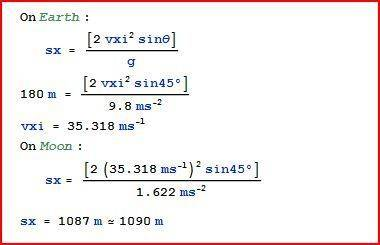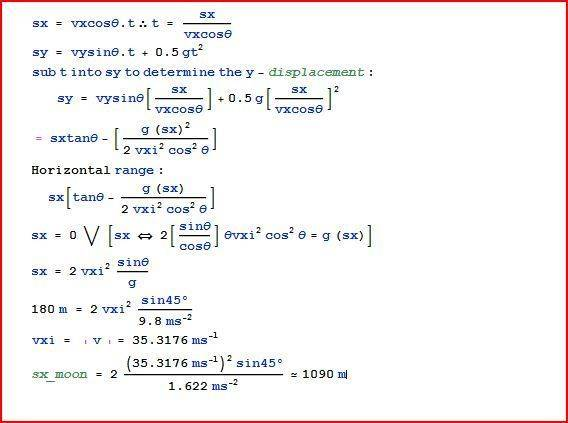How far can a golf ball travel on the moon?

Homework Statement

If you can hit a golf ball 180m on Earth, how far can you hit it on the Moon?

The Attempt at a Solution

Since this question deals with range;

sx = [vxi^2 sinΘ]/g

My problem is that I do not have the velocity at which the golf ball is being hit. As for the launch angle, I could make a probable assumption that the golf ball is launched at a 45°.
Would I be valid to make the assumption the ball would travel 6x further on the Moon than on the Earth given the gravitational pull on the Moon is 1/6 that of Earth?

Last edited:

tiny-tim
Homework Helper
hi negation!Would I be valid to make the assumption the ball would travel 6x further on the Moon than on the Earth given the gravitational pull on the Moon is 1/6 that of Earth?

negative!

work it out!!
As for the launch angle, I could make a probable assumption that the golf ball is launched at a 45°.

yes, maximum range (for fixed speed) is at 45°•1 person
rude man
Homework Helper
Gold Member
You should calculate the range based on the different values of g on Earth and on the Moon.
Assume arbitrary velocity v and angle theta.

You will find out when you finish your calculations whether the velocity and/or angle impact the answer to the question.

•1 person
haruspex
Homework Helper
Gold Member
sx = vxi^2 sinΘ
You've left a very important term of that equation.
(It's obviously wrong because the dimensions don't match. You have a distance on one side and the square of a velocity on the other.)

You've left a very important term of that equation.
(It's obviously wrong because the dimensions don't match. You have a distance on one side and the square of a velocity on the other.)

I carelessly left out the time variable, t.

haruspex
Homework Helper
Gold Member
I carelessly left out the time variable, t.

No, that's not what's missing. Check where you got that equation from.

No, that's not what's missing. Check where you got that equation from.

It has been corrected: sx = [vxi^2 sinΘ]/g after having derived it from the trajectory equation.

hi negation!Negative!

work it out!!

yes, maximum range (for fixed speed) is at 45°you should calculate the range based on the different values of g on earth and on the moon.
Assume arbitrary velocity v and angle theta.

You will find out when you finish your calculations whether the velocity and/or angle impact the answer to the question.

you've left a very important term of that equation.
(it's obviously wrong because the dimensions don't match. You have a distance on one side and the square of a velocity on the other.)haruspex
Homework Helper
Gold Member
It has been corrected: sx = [vxi^2 sinΘ]/g after having derived it from the trajectory equation.
Right, and from that you can see that the distance is inversely proportional to the strength of gravity. So your guess was right: one sixth the gravity means 6 times the distance.

•1 person
ehild
Homework Helper
Since this question deals with range;

sx = [vxi^2 sinΘ]/g

The equation is wrong.

ehild

haruspex
Homework Helper
Gold Member
The equation is wrong.

ehild
Ah yes, good catch. There's a 2 missing, right?

Ah yes, good catch. There's a 2 missing, right?

I noticed. It ought to be sin^2θ

haruspex
Homework Helper
Gold Member
I noticed. It ought to be sin^2θ
Not quite.

ehild
Homework Helper
The time of flight is t=2viy/g=2visin(θ)/g. The horizontal displacement during that time is sx=vicos(θ)t= 2vi2cos(θ)sin(θ)/g=[vi2sin(2θ)]/g. With a given initial speed, maximum range is at θ=45°.

Do not use vix in the formula for sx.

ehild

Not quite.

Are you sure?

I managed to obtain the right answer. I have poor memorizing abilities and as such I derive equations I require(very time consuming).

The formula I derived is x = [2vxi^2sin45°]/g

This enables me to obtain vxi. After which I use the above formula to determine the x-displacement on the moon.

ehild
Homework Helper
I wonder how you got that formula...

ehild

I wonder how you got that formula...

ehildit works fine...

I have used my own arbitrary label of the variables but they essentially implies the same thing.
In my formula, I determine the time taken for the particle to travel from xi to xf.
The time taken for particle to be displaced from xi - xf can be substituted into the y-displacement equation to determine the displacement of the particle along the y-axis-this allows me to determine the x and y coordinate of the particle at a given time.
Simplifying the equation where t = sx/ vicosΘ into the y = visinΘ-0.5gt^2 produces a quadratic equation which allows me to determine the 2 possible x-coordinate of the particle where y = 0 since the trajectory has a parabolic characteristic.

In simplifying further, I obtain x = 2vi^2sinΘ/g; given 3 known variables, I can find the one unknown.

I saw the reasoning in the link you provided. The reasoning in the link begins by using the y-displacement equation to determine the time taken for the particle to be displaced from yi to yi. Then it subs that amount of time into the x-displacement equation to determine the horizontal range the particle travels in that same amount of time.

As for the formula I used, I begin with determining the time taken for the particle to be displaced from xi -xf.

Last edited:
ehild
Homework Helper
I have used my own arbitrary label of the variables but they essentially implies the same thing.

So what are vx and vy (or vxi and vyi) in your derivation?

In my formula, I determine the time taken for the particle to travel from xi to xf.
The time taken for particle to be displaced from xi - xf can be substituted into the y-displacement equation to determine the displacement of the particle along the y-axis-this allows me to determine the x and y coordinate of the particle at a given time.
Simplifying the equation where t = sx/ vicosΘ into the y = visinΘ-0.5gt^2 produces a quadratic equation which allows me to determine the 2 possible x-coordinate of the particle where y = 0 since the trajectory has a parabolic characteristic.

That is correct.

In simplifying further, I obtain x = 2vi^2sinΘ/g;

That is still wrong.

ehild

haruspex
Homework Helper
Gold Member
View attachment 65737

it works fine...
The line that start "sx = 0 V" is fine (except for a spurious extra θ), but in going from there to the next line you dropped a cos(θ).
There is an easier way: forget sy, compute the flight time. Time to reach apex is vy/g.

The line that start "sx = 0 V" is fine (except for a spurious extra θ), but in going from there to the next line you dropped a cos(θ).
There is an easier way: forget sy, compute the flight time. Time to reach apex is vy/g.

I saw that. It was a typo but, admittedly, I was lazy to have it changed.
I went through the link again, understood the reasoning, and I must say it has more clarity.

So what are vx and vy (or vxi and vyi) in your derivation?

ehild

Understood.

haruspex
Homework Helper
Gold Member
I saw that. It was a typo but, admittedly, I was lazy to have it changed.
OK, but that's why you got this wrong formula: x = 2vi^2sinΘ/g
What formula do you get now?

OK, but that's why you got this wrong formula: x = 2vi^2sinΘ/g
What formula do you get now?

I got this: x = vi^2sin[2Θ]/g

I made a careless mistake by overlooking the trig identity where 2cos(x)sin(x) = sin(2x):

Last edited: